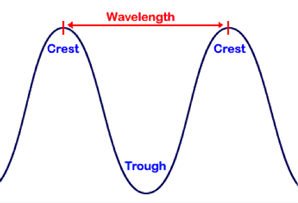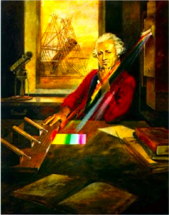Electromagnetic Spectrum

You can replicate Herschel’s famous experiment.

Here is how.

Hertz,

Hz = cycle/sec

Equation

f = c/λ

6.1

Wavelength and FrequencyThe wavelength of light is just the measure of the distance between two wave crests or two wave troughs. The units of measurement often vary depending on the wavelength region one is discussing. For example, radio waves have the longest wavelengths and scientists often use centimeters or even meters to describe their wavelength.

Visible light is often measured in units of nanometers (nm), one-millionth of a meter). Infrared light is commonly measured in microns, and x-rays are often measured in angstroms (one-ten millionth of a millimeter). To avoid having to know how big all these different units are it is convenient just to use fractions of a meter for all of them. But the fraction is so tiny – a billionth, or trillionth or much smaller – that scientific notation is used with a minus in front of the exponent. For example, a millionth of a meter is 10-6, a trillionth is 10-12 and a quadrillionth is 10-15!

Frequency is the number of waves per second; very high frequency waves such as gamma rays vibrate up and down at the amazing rate of 1020 times per second. A lot faster than humming bird wings flap (about 50 times per second)!  The unit to measure these vibrations is the hertz which is the unit for frequency: 1 Hz= 1 cycle/sec.

Visible Light

The part of the EMS that we are most familiar with is visible light. It is the only part of the EMS we can see, so it makes up our visual world. Here is a clever little widget that shows the exact color of any wavelength of visible light. To be a smart-alec, you could say you’d like to paint your room with paint at the wavelength of 517 nm. Nobody would understand you except a physicist and they don’t usually paint rooms.

The Greek letter lambda, λ, is the symbol for wavelength. The frequency, f, of a wave is defined as how many of the peaks pass a certain point in a given time. Since all radiation travels at the speed of light, c, we can then say:

frequency = speed of light divided by wavelength
f = c/λ

Sometimes the Greek symbol, “ν”, pronounced “new”, is used instead of “f”. So;

ν = c/λ

Now when someone asks you; What’s new?, you’ll know what to tell them!

Did you notice the important fact that frequency and wavelength are reciprocals of each other? If you know one you can use Eqn 6.1 to convert it to the other.

Diagram: NASAMethane (CH4) is a common gas found in planetary atmospheres of Earth, Mars, all the Jovian planets, and Saturn’s moon, Titan. On Earth, it is produced by both chemical and biological processes, so it could be a biomarker for life on other worlds! Methane has a characteristic spectral line at 7.66 nm. What is its frequency?

hertz

First, we have to change 7.66 nm to units we understand! As said above “nm” stands for nanometer, which is one billionth of a meter, so 7.66 nm = 7.66 x 10-9 meters. f = c/λ = 3 x 108 m/s / 7.66 x 10-9 m = 3.9 x 1016 /sec = 3.9 x 1016 hertz

The light for methane is really beating fast! Look back at the EMS graphic above and you’ll see that a frequency of 1016 cycles/sec is near the middle of the ultraviolet (UV). To detect the 7.66 μm CH4 spectral line requires observing in the ultraviolet, a part of the spectrum that is blocked by the Earth’s atmosphere so space telescopes are required.

Radio waves, which have the lowest frequency, oscillate "only" 1 million times each second, and at the high frequency end of the EMS, gamma rays vibrate up and down 1020 times a second. These rates are very different from sound waves, which are typically at hundreds to thousands of vibrations per second. The physics of electromagnetism is something we can calculate but the rates and sizes of waves are beyond our normal experiences. Except that we do see light, and we detect UV with our skin – too much UV and we get sunburned.A very important emission line of neutral hydrogen lies at 21 cm, in the radio part of the EMS. This line is used to probe our galaxy’s interstellar gases, detect spiral arms and measure their rotation rate. What is the frequency associated with 21 cm?

hertz

f = c/λ = 3 x 108 m/s / 2.1 x 10-1 m = 1.4 x 109 hertzDistant spiral galaxy image - NASA - Hubble Space TelescopeThe first indication that there was more than just visible light came in 1800 when the astronomer William Herschel was experimenting with the temperature of different frequencies of light. He laid out a series of thermometers behind a prism that broke the sun’s light into a rainbow of colors. He positioned the thermometers so that the different colors of light from the prism would fall on them. He then recorded the temperature of each thermometer. One thermometer was outside the range of the spectrum cast by the prism. It sat just beyond the edge of the red part of the spectrum. Herschel wanted to use this thermometer as a control data point showing what the temperature would read if no light fell on the thermometer. To his great surprise, that thermometer recorded a temperature higher than any of the others! There must be invisible light beyond the red part of the spectrum. Herschel had discovered infrared radiation.